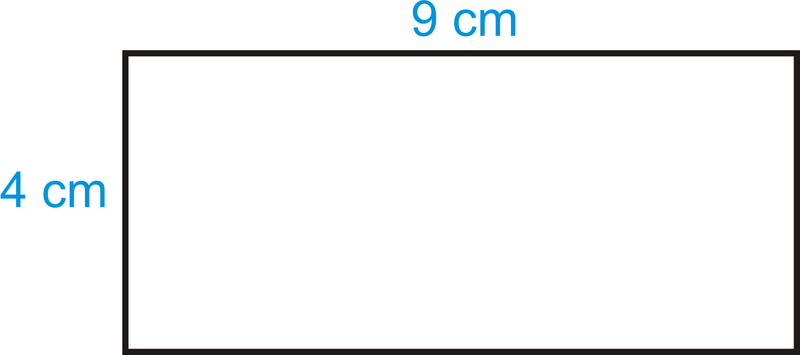## How to Calculate and Solve for the Area, Width and Length of a Rectangle | The Calculator EncyclopediaThe image above is a rectangle with a length of 9 cm and a width of 4 cm.
To compute the area of a rectangle requires two essential parameters which are the length of the rectangle and the width of the rectangle.

The formula for calculating the area of a rectangle is:

A = lw

Where:

A = Area of a Rectangle
l = Length of a Rectangle
w = Width of a Rectangle

Let’s solve an example
Find the area of a rectangle where the length of the rectangle is 9 cm and the width of the rectangle is 4 cm.

This implies that:
l = Length of the Rectangle = 9 cm
w = Width of the Rectangle = 4 cm

A = lw
A = (9)(4)
A = 36

Therefore, the area of the rectangle is 36 cm2.

How to Calculate the Length of a Rectangle when the Area and Width of the Rectangle is Given

The formula for calculating the length of the rectangle is:

l = A / w

Where:

l = Length of the Rectangle
A = Area of the Rectangle
w = Width of the Rectangle

Let’s solve an example:
Find the length of a rectangle where the area of the rectangle is 45 cm2 and the width of the rectangle is 9 cm.

This implies that:
A = Area of the Rectangle = 45 cm2
w = Width of the Rectangle = 9 cm

l = A / w
l = 45 / 9
l = 5

Therefore, the length of the rectangle is 5 cm.

How to Calculate the Width of a Rectangle when the Area and Length of the Rectangle is Given

The formula for calculating the width of the rectangle is:

w = A / l

Where:

w = Width of the Rectangle
A = Area of the Rectangle
l = Length of the Rectangle